# LiquidMotor Class Usage#

Here we explore different features of the LiquidMotor class.

## Creating a Liquid Motor#

To define a liquid motor, we will need a few information about our motor:

• The thrust source file, which is a file containing the thrust curve of the motor. This file can be a .eng file, a .rse file, or a .csv file. See more details in Thrust Source Details

• Tank objects, which will define propellant tanks. See more details in Tank Usage

• Position related parameters, such as the position of the center of mass of the dry mass, the position of the center of mass of the grains, and the position of the nozzle. See more details in Motor Coordinate Systems

Let’s first import the necessary modules:

```from math import exp
from rocketpy import Fluid, LiquidMotor, CylindricalTank, MassFlowRateBasedTank
```

Then we must first define the tanks:

Tank Usage

```# Define fluids
oxidizer_liq = Fluid(name="N2O_l", density=1220)
oxidizer_gas = Fluid(name="N2O_g", density=1.9277)
fuel_liq = Fluid(name="ethanol_l", density=789)
fuel_gas = Fluid(name="ethanol_g", density=1.59)

# Define tanks geometry
tanks_shape = CylindricalTank(radius = 0.1, height = 1.2, spherical_caps = True)

# Define tanks
oxidizer_tank = MassFlowRateBasedTank(
name="oxidizer tank",
geometry=tanks_shape,
flux_time=5,
initial_liquid_mass=32,
initial_gas_mass=0.01,
liquid_mass_flow_rate_in=0,
liquid_mass_flow_rate_out=lambda t: 32 / 3 * exp(-0.25 * t),
gas_mass_flow_rate_in=0,
gas_mass_flow_rate_out=0,
liquid=oxidizer_liq,
gas=oxidizer_gas,
)

fuel_tank = MassFlowRateBasedTank(
name="fuel tank",
geometry=tanks_shape,
flux_time=5,
initial_liquid_mass=21,
initial_gas_mass=0.01,
liquid_mass_flow_rate_in=0,
liquid_mass_flow_rate_out=lambda t: 21 / 3 * exp(-0.25 * t),
gas_mass_flow_rate_in=0,
gas_mass_flow_rate_out=lambda t: 0.01 / 3 * exp(-0.25 * t),
liquid=fuel_liq,
gas=fuel_gas,
)
```
```Warning: Adding spherical caps to the tank will not modify the total height of the tank 1.2 m. Its cylindrical portion height will be reduced to 1.0 m.
```

Note

Here we define two tanks, one for the oxidizer and one for the fuel. We use the MassFlowRateBasedTank, which means that the mass flow rates of the liquid and gas are defined by the user.

In this case, we are using a lambda functions to define the mass flow rates, but .csv files can also be used. See more details in `rocketpy.motors.Tank.MassFlowRateBasedTank.__init__`

Now we can define our liquid motor and add the tanks. We are using a lambda function as the thrust curve, but keep in mind that you can use different formats here.

```example_liquid = LiquidMotor(
thrust_source=lambda t: 4000 - 100 * t**2,
dry_mass=2,
dry_inertia=(0.125, 0.125, 0.002),
center_of_dry_mass_position=1.75,
nozzle_position=0,
burn_time=5,
coordinate_system_orientation="nozzle_to_combustion_chamber",
)
```

Caution

Pay special attention to:

• `dry_inertia` is defined as a tuple of the form `(I11, I22, I33)`. Where `I11` and `I22` are the inertia of the dry mass around the perpendicular axes to the motor, and `I33` is the inertia around the motor center axis.

• `dry inertia` is defined in relation to the center of dry mass, and not in relation to the coordinate system origin.

• `center_of_dry_mass_position`, `nozzle_position` and the tanks `position` are defined in relation to the coordinate system origin, which is the nozzle outlet in this case.

• Both `dry_mass` and `center_of_dry_mass_position` must consider the mass of the tanks.

You can find details on each of the initialization parameters in `rocketpy.LiquidMotor.__init__`

After defining the motor, we can plot basic attributes using the `info()` method.

```example_liquid.info()
```
```Nozzle Details

Motor Details
Total Burning Time: 5 s
Total Propellant Mass: 53.020 kg
Average Propellant Exhaust Velocity: 327.234 m/s
Average Thrust: 3166.493 N
Maximum Thrust: 4000.0 N at 0.0 s after ignition.
Total Impulse: 15832.466 Ns

```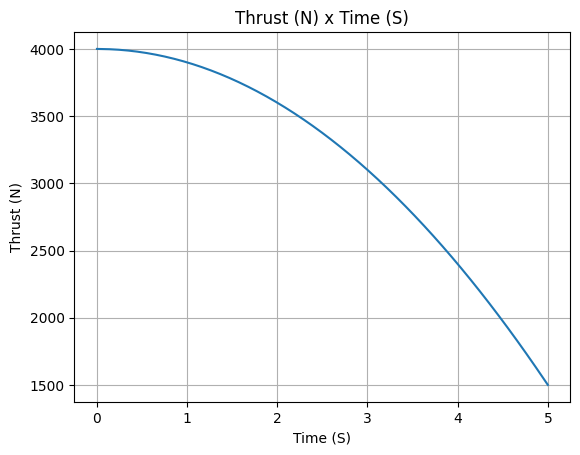Other plots can also be done, in order to check if the motor is behaving as expected. For example:

• Propellant mass

• Mass flow rate

• Motor center of mass

• Inertial moment

• Exhaust velocity

```example_liquid.propellant_mass.plot(0, 5)
``````example_liquid.mass_flow_rate.plot(0, 5)
```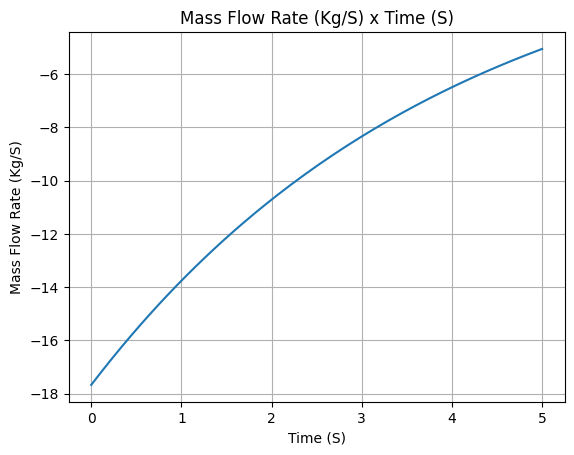```example_liquid.center_of_mass.plot(0, 5)
```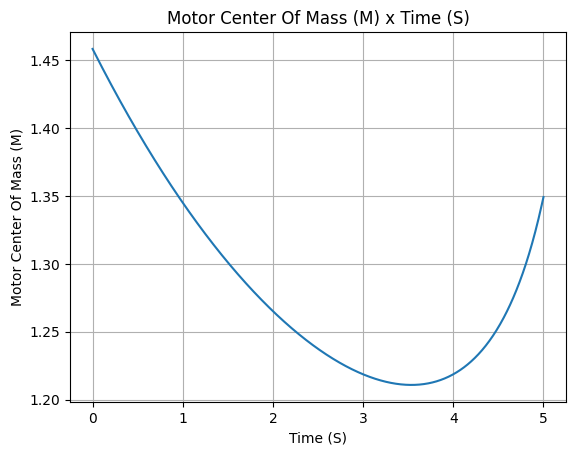```example_liquid.I_11.plot(0, 5)
```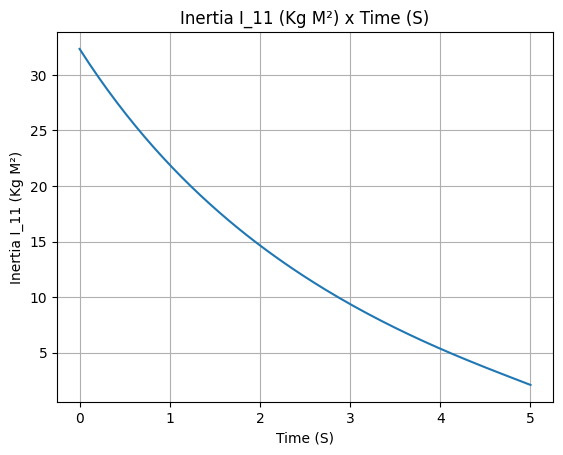```example_liquid.exhaust_velocity.plot(0, 5)
```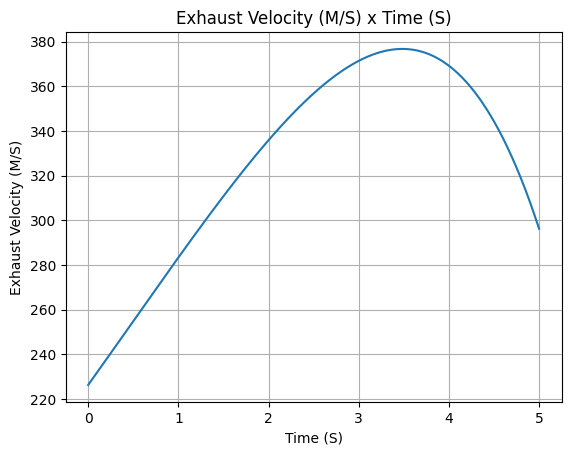Alternatively, you can plot all the information at once:

```example_liquid.all_info()
```
```Nozzle Details